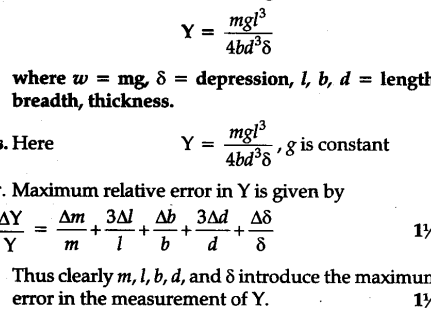# Point out the measurable likely to create the maximum error in die following experimental measurement: Young's Modulus' Y' of the material of the beam is calculated using the relation

Point out the measurable likely to create the maximum error in die following experimental measurement: Young’s Modulus’ Y’ of the material of the beam is calculated using the relation